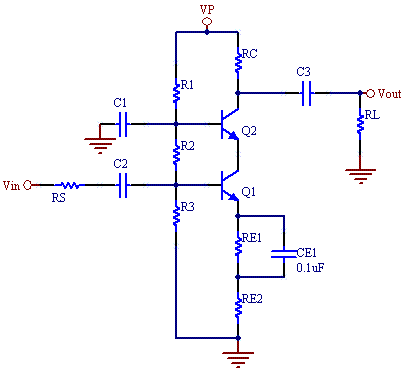# BJT Cascode Amplifier Calculator

This calculator computes bias voltage and current levels, as well as gain and frequency response for the Cascode amplifier.

The cascode amplifier has high gain and high band width. It overcomes the Miller capacitance limitations of the common emitter amplifier by using a second transistor as common- base current buffer. As a result it can achieve gain bandwidths orders of magnitude larger than the common emitter amplifiers. The only cost is that a second transistor is needed, and a higher voltage supply is needed so that the transistors have decent operating margins.

For the this cascode gain is set by the ratio of RC/RE2. To increase bandwidth maximize the sum of RE1 and RE2.It should be noted, that in the real world, for frequencies above 2MHz, a second emitter follower buffer stage is needed to prevent loading of the output stage, and reduced frequency response. The capacitance from even a 1MHz scope probe will roll the frequency of this amplifier off at 2 MHz. To measure frequency response on a scope, measure on the output of the cascode through a buffer.

 R1 (K ohms) R2 (K ohms) R3 (K ohms) RC (Collector resistor) (K ohms) RE1 (Emitter resistor, AC bypassed) (K ohms) RE2 (Emitter resistor, gain control) (ohms) VP (Supply Voltage) (V) Beta (DC Current Gain) VBE (Base to emitter drop) (V) Rs (Source Resistance): (ohms) RL (Load resistor) (ohms) fT (Current Gain BW Product): (MHz) CCB (Cu Collector-Base Cap.): (pF) CBE (Cπ, Base-Emitter Cap.): Optional (pF) VC1 (Collector Voltage) (V) VE1 (Emitter Voltage) (V) VB1 (Base Voltage) (V) VC2 (Collector Voltage) (V) VE2 (Emitter Voltage) (V) VB2 (Base Voltage) (V) IC (Collector Voltage) (mA) IB (Base Voltage) (mA) gm (Transconductance) rπ(Input Resistance of BJT at low freq) (ohms) RIN (Input Resistance of Amp) (K ohms) A (Amplifier Voltage Gain) f1 (MHz) f2 (MHz) BW (MHz)

### Equations

VB1= R3/(R1+R2+R3)

VB2= (R2+R3)/(R1+R2+R3)

VE1= VB1-VBE

VE2= VB2-VBE

VC1= VE2

VC2= VP - IC*RC

RB1= R3*(R1+R2)/ (R1+R2+R3)

IB*RB1 + IB*Beta*RE+VBE=VB1

IB= (VB1-VBE)/(RB1+Beta*RE)

IC= Beta*IB

gm = Ic/25mA

rπ=Beta/gm

If RE2 is zero ohms:

A= -gm*((RL||RC)*(R2||R3))/(R23+RS)*(rπ/(rπ+Rx+(R2||R3||RS)),

else

A= -(RL||RC)/RE2

Rx is typically10-30 ohms, we use 20 in the calculator.

fT= gm/(2π*(CBE+CBC))

f1= 1/(Rin*(Cbe+ 2*Ccb))/(2*π);

f2= 1/(RL*Ccb)/(2*π);

The lower pole is dominate:

BW= f1;